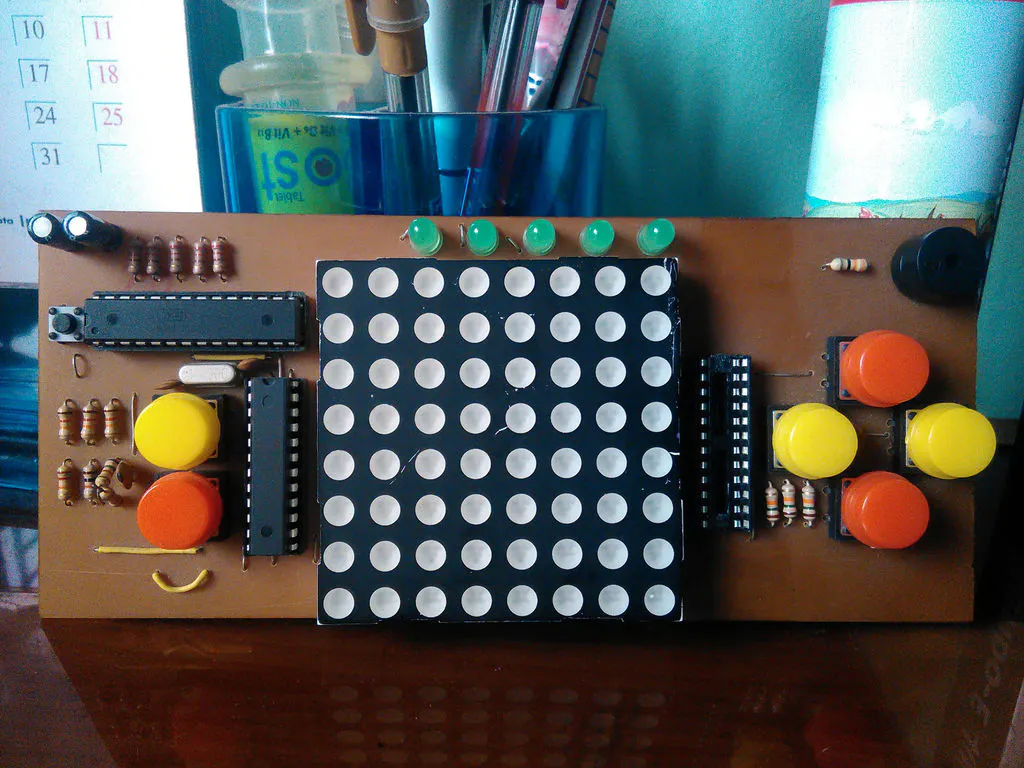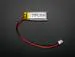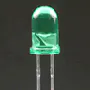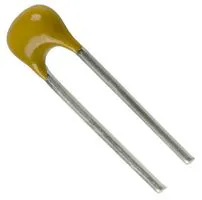# Arduino Dot Matrix Game Console

A DIY Game console you can make and upload your own game like Snake or Pong.

IntermediateFull instructions provided15 hours15,978## Things used in this project

### Hardware componentsArduino UNO
×1Li-Ion Battery 100mAh
×1
 ATmega328P Microcontroller
×1
 MAX7219CNG
×2
 2.379" Bi-color Dot Matrix Display
×1
 Push Buttons or Tactile Switch
×6Buzzer
×15 mm LED: Green
×5Resistor 10k ohm
×15Capacitor 10 pF
×4Capacitor 1 µF
×2
 IC base
×3
 165mm X 73mm PCB board
×1

### Hand tools and fabrication machinesSoldering iron (generic)Hot glue gun (generic)
 Seeed PCB drill

## Custom parts and enclosures

### PCB Board Layout

Designed in Eagle

## Schematics

### Watch Schematic

Designed in Eagle Layout Editor

## Code

### Source Code for Snake Game

C/C++
Developed in Arduino Environment
```#include "LedControl.h"
// connection to MAX7219 (data, clik, load, #of dispplay)
LedControl lc = LedControl(11,13,10,1);

// direction
const int TOP    = 0;
const int RIGHT  = 1;
const int BOTTOM = 2;
const int LEFT   = 3;
// Snake length
const int MAX_SNAKE_LENGTH = 16;

// Variables
int direction = RIGHT;                               // direction of movement
int snakeX[MAX_SNAKE_LENGTH];                      // X-coordinates of snake
int snakeY[MAX_SNAKE_LENGTH];                      // Y-coordinates of snake
int snakeLength = 3;
int score = 3;//
unsigned long prevTime = 0;                        // for gamedelay (ms)
unsigned long delayTime = 500;                     // Game step in ms

int fruitX, fruitY;
unsigned long fruitPrevTime = 0;
unsigned long bonusTime = 0;
unsigned long bonusPrevTime = 0;
int fruitLed = true;
int bonusLed = true;
int bonusX, bonusY;

void setup(){
////wake up the MAX72XX from power-saving mode
lc.shutdown(0,false);
//set a medium brightness for the Leds
lc.setIntensity(0, 15);
//Switch all Leds on the display off.
lc.clearDisplay(0);
Serial.begin(9600);

pinMode(0,INPUT);
//pinMode(17,INPUT);
pinMode(1,INPUT);
pinMode(2,INPUT);
pinMode(3,INPUT);
pinMode(4,INPUT);
pinMode(5,INPUT);
//buzzer
pinMode(12,OUTPUT);

snakeX = 0;
snakeY = 4;
for(int i=1; i<MAX_SNAKE_LENGTH; i++){
snakeX[i] = snakeY[i] = -1;
}
makeFruit();

}

void loop(){
delay(10);
checkButtons();  // if any button is pressed or not

unsigned long currentTime = millis();
if(currentTime - prevTime >= delayTime){
nextstep();

prevTime = currentTime;
}
draw(); // make snack & food
}

void checkButtons(){
direction = TOP;
direction = RIGHT;
direction = LEFT;
direction = BOTTOM;
}

void draw(){
lc.clearDisplay(0);
drawSnake();
drawFruit();

}
void drawSnake(){
for(int i=0; i<snakeLength; i++){
lc.setLed(0,snakeX[i], snakeY[i], true);
}
}
void drawFruit(){
if(inPlayField(fruitX, fruitY)){
unsigned long currenttime = millis();
fruitLed = (fruitLed == true) ? false : true;
fruitPrevTime = currenttime;
}
lc.setLed(0,fruitX, fruitY, fruitLed);
}
}
boolean inPlayField(int x, int y){
return (x>=0) && (x<8) && (y>=0) && (y<8);
}
void nextstep(){
for(int i=snakeLength-1; i>0; i--){
if((direction == RIGHT)&&(snakeX-snakeLength == 7))
snakeX = -1;
else if((direction == LEFT)&&(snakeX+ snakeLength == 0))
snakeX = 8;
else snakeX[i] = snakeX[i-1];
if((direction == TOP) && (snakeY+snakeLength == 0))
snakeY=8;
else if((direction == BOTTOM) && (snakeY-snakeLength == 7))
snakeY=-1;
else snakeY[i] = snakeY[i-1];
}
switch(direction){
case TOP:
snakeY = snakeY-1;
break;
case RIGHT:
snakeX = snakeX+1;
break;
case BOTTOM:
snakeY = snakeY+1;
break;
case LEFT:
snakeX=snakeX-1;
break;
}
if((snakeX == fruitX) && (snakeY == fruitY)){
snakeLength++;
score++;
tone(12,4500,50);
if(snakeLength < MAX_SNAKE_LENGTH){
makeFruit();
}
else {
fruitX = fruitY = -1;
}
if(score%8==0)
{
snakeLength = 3;
delayTime = delayTime - 100;
}
}
snakeItSelf();
}
void makeFruit(){
int x, y;
x = random(0, 8);
y = random(0, 8);
while(isPartOfSnake(x, y)){
x = random(0, 8);
y = random(0, 8);
}
fruitX = x;
fruitY = y;
}

boolean isPartOfSnake(int x, int y){
for(int i=0; i<snakeLength-1; i++){
if((x == snakeX[i]) && (y == snakeY[i])){
return true;
}
}
return false;
}
void snakeItSelf(){  // check if snack touch itself
for(int i=1;i<snakeLength;i++){
if((snakeX == snakeX[i]) && (snakeY == snakeY[i]))
gameOver();
}
}
void gameOver(){
// game over sound
tone(12,1000,100);
delay(100);
tone(12,1500,200);
delay(200);
tone(12,2000,300);
delay(300);
tone(12, 494,500);
delay(500);
lc.clearDisplay(0);
for(int r = 0; r < 8; r++){
for(int c = 0; c < 8; c++){
lc.setLed(0, r, c, HIGH);
delay(50);
}delay(50);
}
delay(300);
score = 3;
snakeLength = 3;
direction = RIGHT;
snakeX=3;
snakeY=4;
delayTime = 500;
loop();
}

void drawBonus(){
if(inPlayField(fruitX, fruitY)){
unsigned long bonusTime = millis();
if(bonusTime - bonusPrevTime >= 300){
bonusLed = (bonusLed == true) ? false : true;
bonusPrevTime = bonusTime;
}
lc.setLed(0,bonusX, bonusY, bonusLed);
}
}
void makeBonus(){
int x, y;
x = random(0, 8);
y = random(0, 8);
while(isPartOfSnake(x, y)){
x = random(0, 8);
y = random(0, 8);
}
bonusX = x;
bonusY = y;
}
```

## Credits

### Md. Khairul Alam

51 projects • 489 followers
Engineer, developer, maker & hacker. Currently working as a faculty at the University of Asia Pacific, Dhaka, Bangladesh.